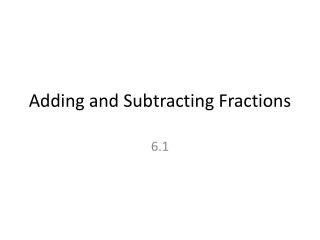DownloadDownload PresentationDownload Presentation- - - - - - - - - - - - - - - - - - - - - - - - - - - E N D - - - - - - - - - - - - - - - - - - - - - - - - - - -
##### Presentation Transcript

1. Common Denominators • Add or subtract the numerators • Keep the denominator • reduce

2. Examples

3. Unlike Denominators • Find the LCM of the denominators (LCD) • Rename the fractions • Add or subtract the numerators • Keep the denominators • reduce

4. Examples

5. Mixed Numbers • Turn into improper fractions

6. Examples:

7. Homework • Page 271 (10-24) all